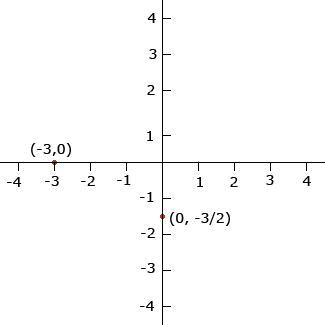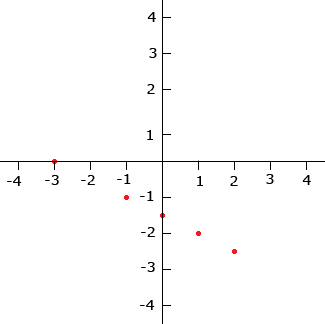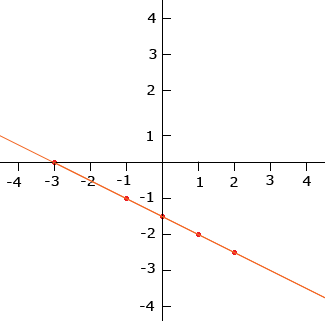Subject: algebra 1 Name: jennifer Who are you: Parent (Secondary) I am having problems explaining how to do these problems to my child . graph:2=x-y graph the equation: 2y+x=-3 can you please help me. Hi Jennifer, I'll look at the second problem. I would start by plotting two special points. First let x = 0 then since 2y + x = -3, when x = 0, 2y + 0 = -3 and hence y = -3/2 . Thus the point x = 0, y = -3/2, in other words the point (0,  -3/2 ) is on the graph. For the second point let y = 0. Then since 2y + x = -3, when y = 0, 0 + x = -3 and hence x = -3. Thus (-3, 0) is on the graph also.Now plot more points, when x = 1, 2y + x = -3 so 2y + 1 = -3 and hence y = -2 when x = -1, 2y + x = -3 so 2y + -1 = -3 and hence y = -1 when x = 2, 2y + x = -3 so 2y + 2 = -3 and hence y = -5/2As you plot more points you can see that they all lie on a straight line and this line is the graph of the equation 2y + x = -3.Now try the other problem. Penny